HomeArtificial IntelligenceArtificial Intelligence DIYTutorial on Self Organizing Maps in R

# Tutorial on Self Organizing Maps in R

 Audio version of the articleSelf-organizing maps (SOMs) are a form of neural network and a wonderful way to partition complex data.  In our lab they’re a routine part of our flow cytometry and sequence analysis workflows, but we use them for all kinds of environmental data (like this).  All of the mainstream data analysis languages (R, Python, Matlab) have packages for training and working with SOMs.  My favorite is the R package Kohonen, which is simple to use but can support some fairly complex analysis through SOMs with multiple data layers and supervised learning (superSOMs).  The Kohonen package has a nice, very accessible paper that describe its key features, and some excellent examples.  This tutorial applies our basic workflow for a single-layer SOM to RGB color data.  RGB color space segmentation is a popular way to evaluate machine learning algorithms, as it is intrinsically multi-variate and inherently meaningful.  Get like colors grouping together and you know that you’ve set things up correctly!

This application of SOMs has two steps.  Each of these steps can be thought of as an independent data reduction step.  It’s important to remember that you’re not reducing dimensions per se, as you would in a PCA, you’re aggregating like data so that you can describe them as convenient units (instead of n individual observations).  The final outcome, however, represents a reduction in dimensionality to a single parameter for all observations (e.g., the color blue instead of (0, 0, 255) in RGB colorspace).  The first step – training the SOM – assigns your observations to map units.  The second step – clustering the map units into classes – finds the structure underlying the values associated with the map units after training.  At the end of this procedure each observation belongs to a map unit, and each map unit belongs to a class.  Thus each observation inherits the class of its associated map unit.  If that’s not clear don’t sweat it.  It will become clear as you go through the procedure.

First, let’s generate some random RGB data.  This takes the form of a three column matrix where each row is a pixel (i.e. an observation).

```#### generate some RGB data ####

## select the number of random RGB vectors for training data

sample.size <- 10000

## generate dataframe of random RGB vectors

sample.rgb <- as.data.frame(matrix(nrow = sample.size, ncol = 3))
colnames(sample.rgb) <- c('R', 'G', 'B')

sample.rgb\$R <- sample(0:255, sample.size, replace = T)
sample.rgb\$G <- sample(0:255, sample.size, replace = T)
sample.rgb\$B <- sample(0:255, sample.size, replace = T)```

Next, we define a map space for the SOM and train the model.  Picking the right grid size for the map space is non-trivial; you want about 5 elements from the training data per map unit, though you’ll likely find that they’re not uniformly distributed.  It’s best to use a symmetrical map unless you have a very small training dataset, hexagonal map units, and a toroidal shape.  The latter is important to avoid edge effects (a toroid has no edges).

One important caveat for the RGB data is that we’re not going to bother with any scaling or normalizing.  The parameters are all on the same scale and evenly distributed between 0 and the max value of 255.  Likely your data are not so nicely formed!

```#### train the SOM ####

## define a grid for the SOM and train

library(kohonen)

grid.size <- ceiling(sample.size ^ (1/2.5))
som.grid <- somgrid(xdim = grid.size, ydim = grid.size, topo = 'hexagonal', toroidal = T)
som.model <- som(data.matrix(sample.rgb), grid = som.grid)```

One you’ve trained the SOM it’s a good idea to explore the output of the `som` function to get a feel for the different items in there.  The output takes the form of nested lists.  Here we extract a couple of items that we’ll need later, and also create a distance matrix of the map units.  We can do this because the fundamental purpose of map units is to have a codebook vector that mimics the structure of the training data.  During training each codebook vector is iteratively updated along with its neighbors to match the training data.  After sufficient iterations the codebook vectors reflect the underlying structure of the data.

```## extract some data to make it easier to use

som.events <- som.model\$codes[]
som.events.colors <- rgb(som.events[,1], som.events[,2], som.events[,3], maxColorValue = 255)
som.dist <- as.matrix(dist(som.events))```

Now that we have a trained SOM let’s generate a descriptive plot.  Since the data are RGB colors, if we color the plot accordingly it should be sensible.  For comparison, we first create a plot with randomized codebook vectors.  This represents the SOM at the start of training.

```## generate a plot of the untrained data.  this isn't really the configuration at first iteration, but
## serves as an example

plot(som.model,
type = 'mapping',
bg = som.events.colors[sample.int(length(som.events.colors), size = length(som.events.colors))],
keepMargins = F,
col = NA,
main = '')```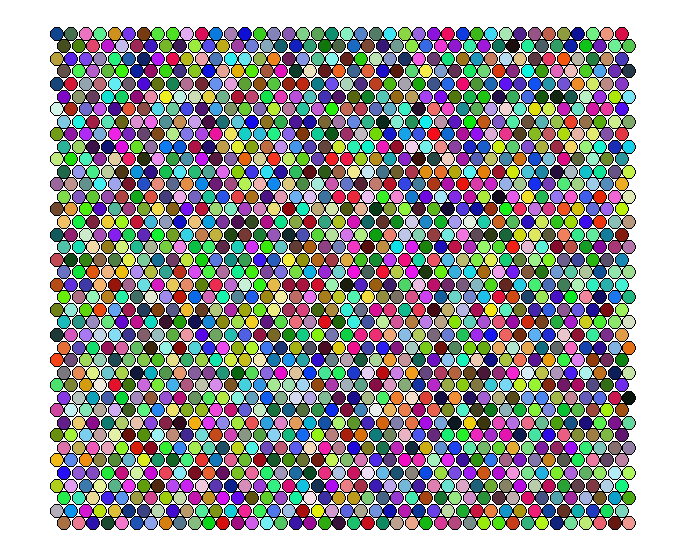And now the trained SOM:

```## generate a plot after training.

plot(som.model,
type = 'mapping',
bg = som.events.colors,
keepMargins = F,
col = NA,
main = '')```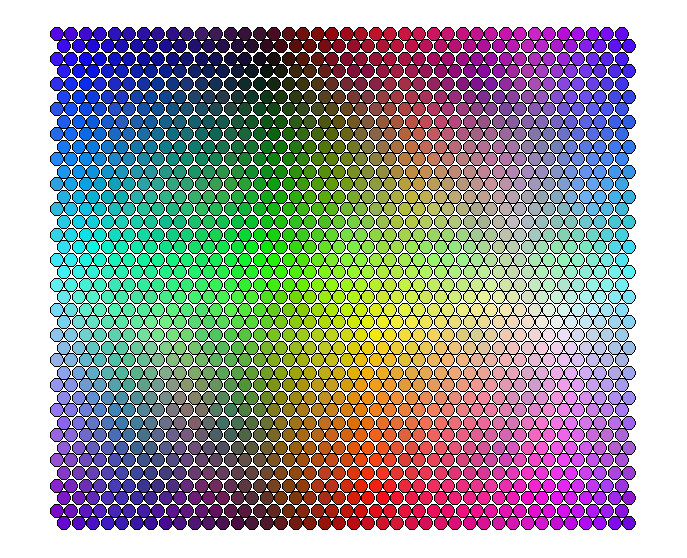So pretty! The next step is to cluster the map units into classes.  As with all clustering analysis, a key question is how many clusters (k) should we define?  One way to inform our decision is to evaluate the distance between all items assigned to each cluster for many different values of k.  Ideally, creating a scree plot of mean within-cluster distance vs. k will yield an inflection point that suggests a meaningful value of k.  In practice this inflection point is extremely sensitive to the size of the underlying data (in this case, the number of map units), however, it can be a useful starting place.  Consider that the RGB data were defined continuously, meaning that there is no underlying structure!  Nonetheless we still get an inflection point.

```#### look for a reasonable number of clusters ####

## Evaluate within cluster distances for different values of k.  This is
## more dependent on the number of map units in the SOM than the structure
## of the underlying data, but until we have a better way...

## Define a function to calculate mean distance within each cluster.  This
## is roughly analogous to the within clusters ss approach

clusterMeanDist <- function(clusters){
cluster.means = c()

for(c in unique(clusters)){
temp.members <- which(clusters == c)

if(length(temp.members) > 1){
temp.dist <- som.dist[temp.members,]
temp.dist <- temp.dist[,temp.members]
cluster.means <- append(cluster.means, mean(temp.dist))
}else(cluster.means <- 0)
}

return(mean(cluster.means))

}

try.k <- 2:100
cluster.dist.eval <- as.data.frame(matrix(ncol = 3, nrow = (length(try.k))))
colnames(cluster.dist.eval) <- c('k', 'kmeans', 'hclust')

for(i in 1:length(try.k)) {
cluster.dist.eval[i, 'k'] <- try.k[i]
cluster.dist.eval[i, 'kmeans'] <- clusterMeanDist(kmeans(som.events, centers = try.k[i], iter.max = 20)\$cluster)
cluster.dist.eval[i, 'hclust'] <- clusterMeanDist(cutree(hclust(vegdist(som.events)), k = try.k[i]))
}

plot(cluster.dist.eval[, 'kmeans'] ~ try.k,
type = 'l')

lines(cluster.dist.eval[, 'hclust'] ~ try.k,
col = 'red')

legend('topright',
legend = c('k-means', 'hierarchical'),
col = c('black', 'red'),
lty = c(1, 1))```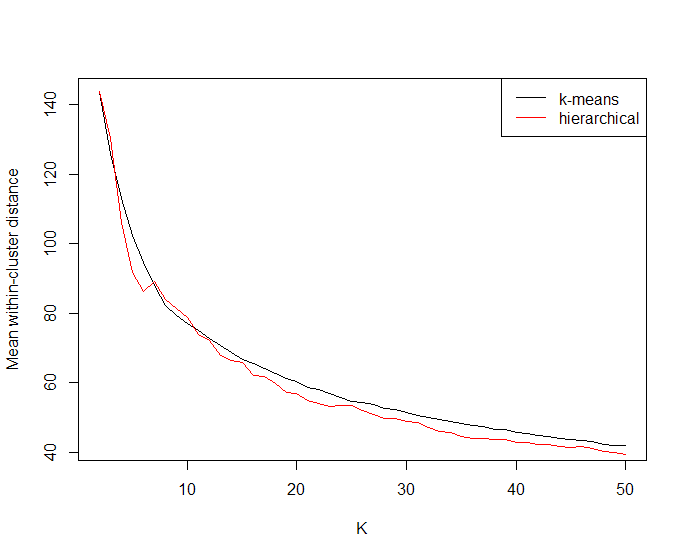Having picked a reasonable value for k (let’s say k = 20) we can evaluate different clustering algorithms.  For our data k-means almost always performs best, but you should choose what works best for your data.  Here will evaluate k-means, hierarchical clustering, and model-based clustering.  What we’re looking for in the plots is a clustering method that produces contiguous classes.  If classes are spread all across the map, then the clustering algorithm isn’t capturing the structure of the SOM well.

```#### evaluate clustering algorithms ####

## Having selected a reasonable value for k, evaluate different clustering algorithms.

library(pmclust)

## Define a function for make a simple plot of clustering output.
## This is the same as previousl plotting, but we define the function
## here as we wanted to play with the color earlier.

plotSOM <- function(clusters){
plot(som.model,
type = 'mapping',
bg = som.events.colors,
keepMargins = F,
col = NA)

}

## Try several different clustering algorithms, and, if desired, different values for k

cluster.tries <- list()

for(k in c(20)){

## model based clustering using pmclust

som.cluster.pm.em <- pmclust(som.events, K = k, algorithm = 'em')\$class # model based
som.cluster.pm.aecm <- pmclust(som.events, K = k, algorithm = 'aecm')\$class # model based
som.cluster.pm.apecm <- pmclust(som.events, K = k, algorithm = 'apecm')\$class # model based
som.cluster.pm.apecma <- pmclust(som.events, K = k, algorithm = 'apecma')\$class # model based
som.cluster.pm.kmeans <- pmclust(som.events, K = k, algorithm = 'kmeans')\$class # model based

## k-means clustering

som.cluster.k <- kmeans(som.events, centers = k, iter.max = 100, nstart = 10)\$cluster # k-means

## hierarchical clustering

som.dist <- dist(som.events) # hierarchical, step 1
som.cluster.h <- cutree(hclust(som.dist), k = k) # hierarchical, step 2

## capture outputs

cluster.tries[[paste0('som.cluster.pm.em.', k)]] <- som.cluster.pm.em
cluster.tries[[paste0('som.cluster.pm.aecm.', k)]] <- som.cluster.pm.aecm
cluster.tries[[paste0('som.cluster.pm.apecm.', k)]] <- som.cluster.pm.apecm
cluster.tries[[paste0('som.cluster.pm.apecma.', k)]] <- som.cluster.pm.apecma
cluster.tries[[paste0('som.cluster.pm.kmeans.', k)]] <- som.cluster.pm.kmeans
cluster.tries[[paste0('som.cluster.k.', k)]] <- som.cluster.k
cluster.tries[[paste0('som.cluster.h.', k)]] <- som.cluster.h
}

## Take a look at the various clusters.  You're looking for the algorithm that produces the
## least fragmented clusters.

plotSOM(cluster.tries\$som.cluster.pm.em.20)
plotSOM(cluster.tries\$som.cluster.pm.aecm.20)
plotSOM(cluster.tries\$som.cluster.pm.apecm.20)
plotSOM(cluster.tries\$som.cluster.pm.apecma.20)
plotSOM(cluster.tries\$som.cluster.k.20)
plotSOM(cluster.tries\$som.cluster.h.20)```

For brevity I’m not showing the plots produced for all the different clustering algorithms. For these data the k-means and hierarchical clustering algorithms both look pretty good, I have a slight preference for the k-means version: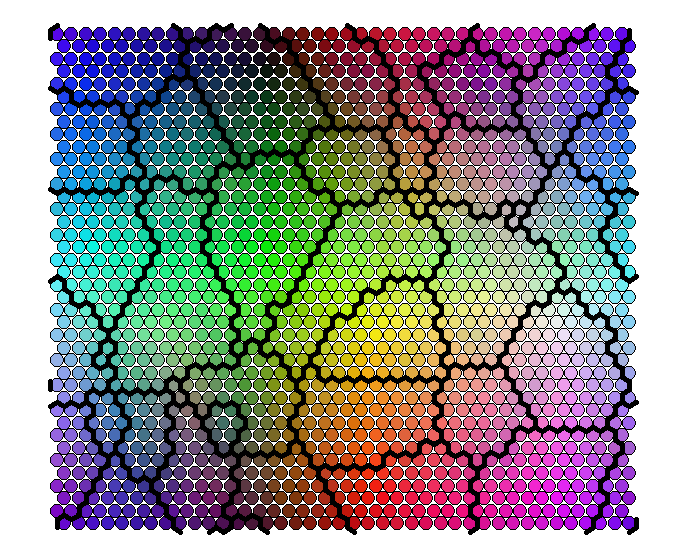The SOM and final map unit clustering represent a classification model that can be saved for use with later data.  Once huge advantage to using SOMs over other analysis methods (e.g., ordination techniques) is their usefulness for organizing newly collected data.  New data, if necessary scared and normalized in the same way as the training data, can be classified by finding the map unit with the minimum distance to the new observation.  To demonstrate this, we’ll generate and classify a small new RGB dataset (in reality classifying in this way is very efficient, and could accommodate a huge number of new observations).  First, we save the SOM and final clustering.

```## The SOM and map unit clustering represent a classification model.  These can be saved for
## later use.

som.cluster <- som.cluster.k
som.notes <- c('Clustering based on k-means')

save(file = 'som_model_demo.Rdata', list = c('som.cluster', 'som.notes', 'som.model', 'som.events.colors'))```

Then we generate new RGB data, classify it, and make a plot to compare the original data, the color of the winning map unit, and the color of the cluster that map unit belongs to.

```#### classification ####

## make a new dataset to classify ##

new.data.size <- 20
new.data <- as.data.frame(matrix(nrow = new.data.size, ncol = 3))
colnames(new.data) <- c('R', 'G', 'B')

new.data\$R <- sample(0:255, new.data.size, replace = T)
new.data\$G <- sample(0:255, new.data.size, replace = T)
new.data\$B <- sample(0:255, new.data.size, replace = T)

## get the closest map unit to each point

new.data.units <- map(som.model, newdata = data.matrix(new.data))

## get the classification for closest map units

new.data.classes <- som.cluster[new.data.units\$unit.classif]

## compare colors of the new data, unit, and class, first define a function
## to calculate the mean colors for each cluster

clusterMeanColor <- function(clusters){
cluster.means = c()
som.codes <- som.model\$codes[]

for(c in sort(unique(clusters))){
temp.members <- which(clusters == c)

if(length(temp.members) > 1){
temp.codes <- som.codes[temp.members,]
temp.means <- colMeans(temp.codes)
temp.col <- rgb(temp.means, temp.means, temp.means, maxColorValue = 255)
cluster.means <- append(cluster.means, temp.col)
}else({
temp.codes <- som.codes[temp.members,]
temp.col <- rgb(temp.codes, temp.codes, temp.codes, maxColorValue = 255)
cluster.means <- append(cluster.means, temp.col)
})
}

return(cluster.means)

}

class.colors <- clusterMeanColor(som.cluster)

plot(1:length(new.data\$R), rep(1, length(new.data\$R)),
col = rgb(new.data\$R, new.data\$G, new.data\$B, maxColorValue = 255),
ylim = c(0,4),
pch = 19,
cex = 3,
xlab = 'New data',
yaxt = 'n',
ylab = 'Level')

axis(2, at = c(1, 2, 3), labels = c('New data', 'Unit', 'Class'))

points(1:length(new.data.units\$unit.classif), rep(2, length(new.data.units\$unit.classif)),
col = som.events.colors[new.data.units\$unit.classif],
pch = 19,
cex = 3)

points(1:length(new.data.classes), rep(3, length(new.data.classes)),
col = class.colors[new.data.classes],
pch = 19,
cex = 3)```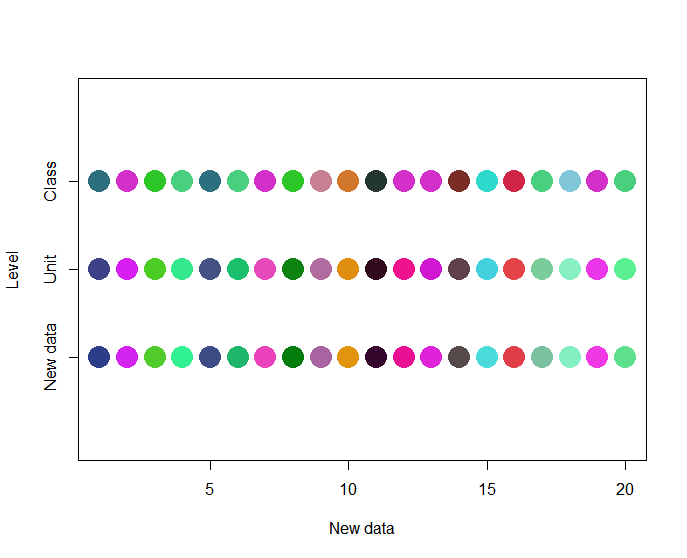Looks pretty good! Despite defining only 20 classes, class seems to be a reasonable representation of the original data. Only slight differences in color can be observed between the data, winning map unit, and class.

- Advertisment -### Can AI Help Us Manifest The Life We Want?

- Advertisment -